Test: Methods of Indeterminate Analysis -2

# Test: Methods of Indeterminate Analysis -2

Test Description

## 10 Questions MCQ Test GATE Civil Engineering (CE) 2023 Mock Test Series | Test: Methods of Indeterminate Analysis -2

Test: Methods of Indeterminate Analysis -2 for Civil Engineering (CE) 2022 is part of GATE Civil Engineering (CE) 2023 Mock Test Series preparation. The Test: Methods of Indeterminate Analysis -2 questions and answers have been prepared according to the Civil Engineering (CE) exam syllabus.The Test: Methods of Indeterminate Analysis -2 MCQs are made for Civil Engineering (CE) 2022 Exam. Find important definitions, questions, notes, meanings, examples, exercises, MCQs and online tests for Test: Methods of Indeterminate Analysis -2 below.
Solutions of Test: Methods of Indeterminate Analysis -2 questions in English are available as part of our GATE Civil Engineering (CE) 2023 Mock Test Series for Civil Engineering (CE) & Test: Methods of Indeterminate Analysis -2 solutions in Hindi for GATE Civil Engineering (CE) 2023 Mock Test Series course. Download more important topics, notes, lectures and mock test series for Civil Engineering (CE) Exam by signing up for free. Attempt Test: Methods of Indeterminate Analysis -2 | 10 questions in 30 minutes | Mock test for Civil Engineering (CE) preparation | Free important questions MCQ to study GATE Civil Engineering (CE) 2023 Mock Test Series for Civil Engineering (CE) Exam | Download free PDF with solutions
 1 Crore+ students have signed up on EduRev. Have you?
Test: Methods of Indeterminate Analysis -2 - Question 1

### A horizontal fixed beam AB of span 6 m has uniform flexural rigidity of 4200 kN m2. During loading, the support B sinks downwards by 25 mm. The moment induced at the end A is

Detailed Solution for Test: Methods of Indeterminate Analysis -2 - Question 1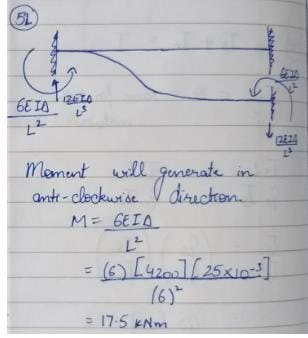Test: Methods of Indeterminate Analysis -2 - Question 2

### A uniform simply supported beam is subjected to a clockwise moment M at the left end. What is the moment required at the right end so that rotation of the right end is zero?

Detailed Solution for Test: Methods of Indeterminate Analysis -2 - Question 2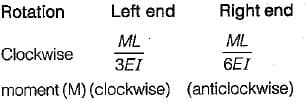at left end
To keep rotation at right and zero a moment should be applied in anticlockwise direction. Let the moment in M'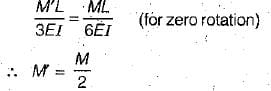Test: Methods of Indeterminate Analysis -2 - Question 3

### The possible direction of sway of the rigid frame shown in figure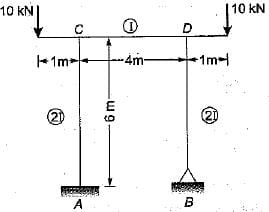Test: Methods of Indeterminate Analysis -2 - Question 4

Four identical beams AE, BE, CE and DEhave been rigidly jointed at E The point O slip and rotates along with member firmly fixed at E.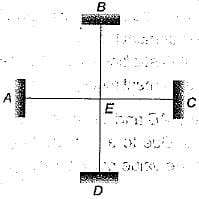Which one among the following is correct?

Detailed Solution for Test: Methods of Indeterminate Analysis -2 - Question 4

Since the member are firmly fixed at E. It is a rigid joint at which all the members will have same rotation. So from slope deflection equations all the members will have moment.

Test: Methods of Indeterminate Analysis -2 - Question 5

Match List-I (Method) with List-lI (Factor) and select the correct answer using the code given below the lists:
List-I
A. Moment distribution method
B. Slope deflection method
C. Kani’s method
D. Force method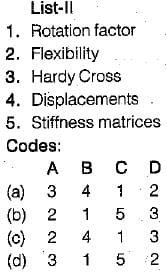Detailed Solution for Test: Methods of Indeterminate Analysis -2 - Question 5

A -3 , B -4 , C - 1 , D - 2

Test: Methods of Indeterminate Analysis -2 - Question 6

Final moment values got through the analysis of a portal frame have been shown in the figure. What is the value of X?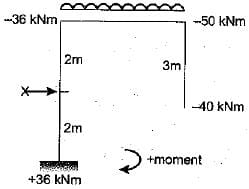Detailed Solution for Test: Methods of Indeterminate Analysis -2 - Question 6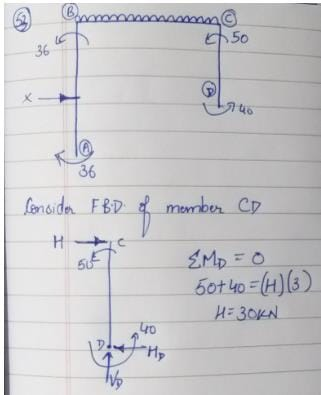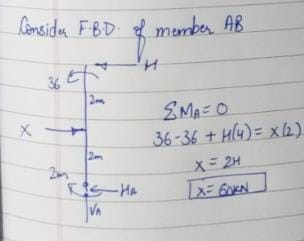Test: Methods of Indeterminate Analysis -2 - Question 7

The figure given above shows a rigid frame. If D . is lateral translation of the joints, slope deflection equation for the member BA be written as: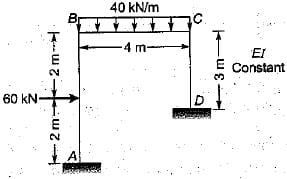Detailed Solution for Test: Methods of Indeterminate Analysis -2 - Question 7

Taking;
- anticlockwise end moment positive
- anticlockwise rotation at member end positive
- anticlockwise chord rotation positive
FEMba = 30 kN-m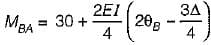Test: Methods of Indeterminate Analysis -2 - Question 8

What are the distribution factors at joint 6 for the members BA and BC respectively, in the figure?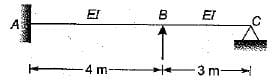Detailed Solution for Test: Methods of Indeterminate Analysis -2 - Question 8

Distribution Factors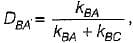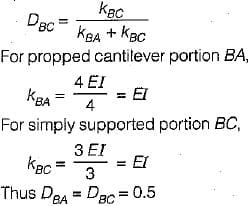Test: Methods of Indeterminate Analysis -2 - Question 9

Which one of the following statements is correct? The principle of superposition is applicable to

Detailed Solution for Test: Methods of Indeterminate Analysis -2 - Question 9

The principle of superposition states that the displacements resulting from each of a number of forces may be added to obtain the displacements resulting from the sum of forces. This method depends upon the linearity of the governing relations between the load and deflection, The linearity depends upon two factors.
(i) the linearity between bending moment and curvature which depends upon the linear elastic materials  In non-linear material superposition of curvatures is not possible.
(ii) the linearity between curvatures and deflection depends upon the assumption that the deflections are so small that the approximate curvature can be used in place of true curvature.

Test: Methods of Indeterminate Analysis -2 - Question 10

Members AB and BC in the figure shown are identical. Due to a moment 2M applied at B, what is the value of axial force in the member AB?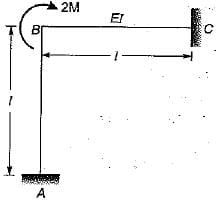Detailed Solution for Test: Methods of Indeterminate Analysis -2 - Question 10

The distribution factors for member AB and SC at S will be 0.5 for both.
Thus member end moment at BC is M.
From carry over factor of 1/2, member end moment at C = M/2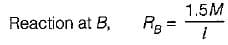This reaction is away from B so axial force in member AB is tensile. This can be found from free body diagram of AB. Similarly axial force in member BC will also be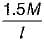but compressive.

## GATE Civil Engineering (CE) 2023 Mock Test Series

25 docs|282 tests
 Use Code STAYHOME200 and get INR 200 additional OFF Use Coupon Code
Information about Test: Methods of Indeterminate Analysis -2 Page
In this test you can find the Exam questions for Test: Methods of Indeterminate Analysis -2 solved & explained in the simplest way possible. Besides giving Questions and answers for Test: Methods of Indeterminate Analysis -2, EduRev gives you an ample number of Online tests for practice

## GATE Civil Engineering (CE) 2023 Mock Test Series

25 docs|282 tests